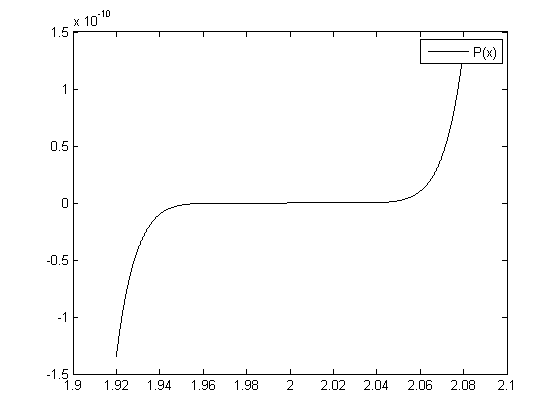# When is your math always wrong?

Last year in my Applied Linear Algebra class we were assigned a homework that introduced Backward Substituation, Forward Substitution, Horner’s Method. Something really interesting happened though as a side effect of the assigned work.

For one of the problems we were given the equation:

```P = @(x) (x-2)^9;
```

Which produces this over [1.92, 2.08]One of the awesome things about MATLAB (or GNU Octave) is that it has a great graphing libraries and a REPL that lets you literally “play” with the problems you are working on. You can visualize it different ways, you can dig deep into different corners, there is really no limit. The net result is a very fun and educational learn-by-doing system very much in the spirit of HtDP. That said I wanted to play around with the function we were given.
Out of curiosity I multiplied out P into PEX:

```PEX = @(x) ...
(x^9)       - (18 * x^8)   + (144 * x^7)  - (672 * x^6) ...
+ (2016 *x^5) - (4032 * x^4) + (5376 * x^3) - (4608 * x^2) ...
+ (2304 * x)  - (512);
```

Now you and I know that P and PEX are equivalent. What do you think will happen we we plot P and PEX together? I figured that we would get one line on top of the other since they are literally the same equation. Why did I want to do this? I can only attribute it to providence.
After thinking about it, go to the next page.

Posted on Categories Article

## 6 thoughts on “When is your math always wrong?”

1.Brad Pollen says:

The world is really digital; it is just that we do not have enough bits. For example, electricity comes in digits called electrons; elements come in digits called atoms, electromagnetic radiation comes in digits called photons….
Stating the obvious, the software solution is based on a normal range of numbers and needed to be better scaled to fit the solution. We humans are not very good at this either. In general, people have gotten use to the idea of a trillion dollar debt, with no real understanding of a trillion. Even examples fail, a trillion seconds is over 32,000 years. 32,000 years is too big to understand. Or in the other direction, carbon dioxide makes up about 390 parts per million by volume of the earth’s atmosphere (or 0.039%). This is too small of number for us to understand without further thought. How do you envision 1 in a million? How about 1 in a trillion? (some pollutants are measured at this level).

2.Nick says:

I think we talked about this before, but I’d be curious what floating point representation is used by Matlab. Is it a Single Precision? Is it possible to do a double precision and try again, or use a Decimal type? These are all different ways of representing floating point numbers in computers, that either use more bytes, or assign more precision to the floating point portion over the integer portion.
Theoretically, each of them would produce a different graph, some with more fidelity to the ideal than others.

3.Grant says:

Such an interesting point; not even sure where to begin on that.

4.Grant says:

Nick:
Tonight I looked into it. I feel like I confirmed that I thought I knew; but am left with more work to understand the numerical representation

5.Anonymous says:

Crazy late followup, hopefully you are notified, but, for the record:
In base b, consider a fraction (reduced, but possibly improper) with denominator D. If the factorization of D is not a subset of the factorization of b, or vice versa, then this fraction cannot be expressed in a finite expansion with a radix point. E.g., factors of 10 are 2 and 5. Then any rational number whose denominator has any number of 2s or 5s as factors—but no others!—can be represented finitely with a decimal point.
Then primorials are the “best” bases, in terms of the number of rationals they can represent finitely with a radix expansion. (2, 6, 30, 210, …)

6.Grant says:

ANONYMOUS:
Thanks.